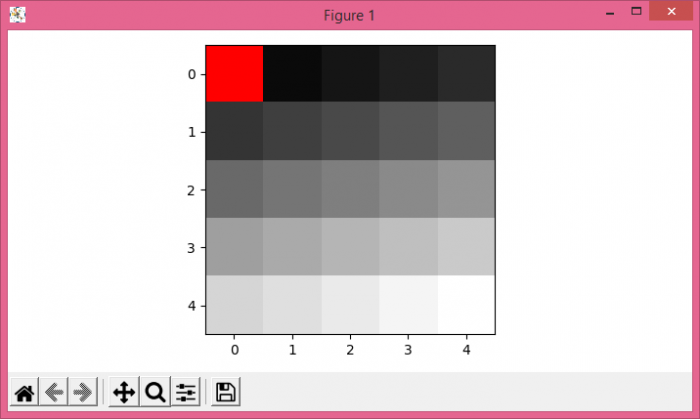# How to redefine a color for a specific value in a Matplotlib colormap?

To redefine a color for a specific value in matplotlib colormap, we can take the following steps −

• Get a colormap instance, defaulting to rc values if *name* is None using get_cmap() method, with gray colormap.

• Set the color for low out-of-range values when "norm.clip = False" using set_under() method.

• Using imshow() method, display data an image, i.e., on a 2D regular raster.

• To display the figure, use show() method.

## Example

import numpy as np
from matplotlib import pyplot as plt, cm
plt.rcParams["figure.figsize"] = [7.00, 3.50]
plt.rcParams["figure.autolayout"] = True
cmap = cm.get_cmap('gray')
cmap.set_under('red')
plt.imshow(np.arange(25).reshape(5, 5),
interpolation='none',
cmap=cmap,
vmin=.001)
plt.show()

## Output